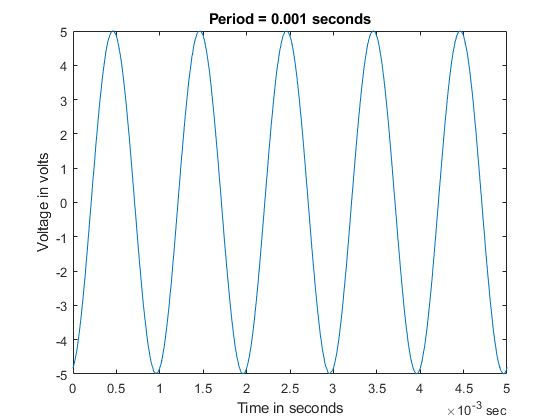# Generate Standard Periodic Waveforms Using Digilent Analog Discovery

Use function generator channels to generate a 1 kHz sinusoidal waveform, and record data at the same time, using an analog input channel.

### Discover Digilent Devices

Discover Digilent devices connected to your system using `daqlist`

```daqlist("digilent") dq = daq("digilent") ```
```ans = 1×4 table DeviceID Description Model DeviceInfo ________ _____________________________________________ ____________________ _______________________ "AD1" "Digilent Inc. Analog Discovery 2 Kit Rev. C" "Analog Discovery 2" [1×1 daq.di.DeviceInfo] dq = DataAcquisition using Digilent Inc. hardware: Running: 0 Rate: 10000 NumScansAvailable: 0 NumScansAcquired: 0 NumScansQueued: 0 NumScansOutputByHardware: 0 RateLimit: [] Show channels Show properties and methods ```

### Add a Function Generator Channel

Add a function generator channel with device ID `AD1` and channel ID `1`. Set the waveform type to `Sine`.

```ch_fgen = addoutput(dq, "AD1", "1", "Sine"); ```

### Set Channel Properties

Set channel gain to 5 (sets the amplitude of the sinusoid to 5 V). Assign the gain to a variable.

```ch_fgen.Name = "AD1_1_fgen" gain = 5; ch_fgen.Gain = gain; ```
```ch_fgen = Index Type Device Channel Measurement Type Range Name _____ ______ ______ _______ ________________ ____________________ ____________ 1 "fgen" "AD1" "1" "Sine" "-5.0 to +5.0 Volts" "AD1_1_fgen" ```

Set the signal frequency to 1 kHz

```ch_fgen.Frequency = 1000; ```

Add an analog input channel with device ID `AD1` and channel ID `1`. Set the measurement type to `Voltage`.

```ch_in = addinput(dq, "AD1", "1", "Voltage"); ch_in.Name = "AD1_1_in" ```
```ch_in = Index Type Device Channel Measurement Type Range Name _____ ____ ______ _______ ________________ __________________ __________ 1 "ai" "AD1" "1" "Voltage (Diff)" "-25 to +25 Volts" "AD1_1_in" ```

### Set DataAcquisition Properties

Acquire data at a higher scan rate than the highest frequency in the generated waveform.

```dq.Rate = 100 * ch_fgen.Frequency; ```

### Generate a Periodic Waveform and Record Input

```[data, startTime] = read(dq, seconds(1)); ```

### Plot Data

```period = 1/ch_fgen.Frequency; plot(data.Time, data.AD1_1_in); xlabel('Time in seconds'); ylabel('Voltage in volts'); title(['Period = ', num2str(period), ' seconds']) xlim([seconds(0) seconds(5*period)]); ylim([-gain gain]); ```